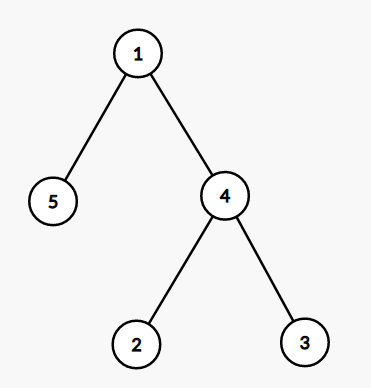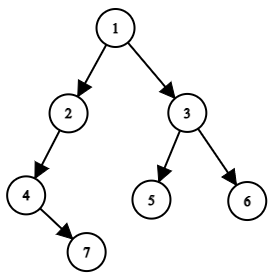New update is available. Click here to update.
Last Updated: 8 Apr, 2021
##### Pairs Violating BST
Moderate
Problem statement#### BST tree has the following properties:-

``````1. For every node, it is greater than or equal to its left child and less than or equal to its right child.
2. Node is greater than equal to the maximum value of its left subtree and less than the minimum value of its right subtree.
3. The maximum in the left sub-tree must be less than equal to the minimum in the right subtree.
``````
##### For example in the following tree:-``````The numbers of pairs violating BST property are:-

(1,5) : 1 should be greater than its left child value.

(4,3) : 4 should be less than its right child value.

(5,4),(5,3):  Maximum of left subtree of 1 is 5 greater
than 2 and 3 of its right subtree.
``````
##### Input Format:
``````The first line contains an integer 'T' which denotes the number of test cases. Then the test cases are as follows.

The first line of each test case contains elements of the tree in the level order form. The line consists of values of nodes separated by a single space. In case a node is null, we take -1 in its place.
````````````For example, the input for the tree depicted in the above image would be :

1
2 3
4 -1 5 6
-1 7 -1 -1 -1 -1
-1 -1
``````

#### Explanation :

``````Level 1 :
The root node of the tree is 1

Level 2 :
Left child of 1 = 2
Right child of 1 = 3

Level 3 :
Left child of 2 = 4
Right child of 2 = null (-1)
Left child of 3 = 5
Right child of 3 = 6

Level 4 :
Left child of 4 = null (-1)
Right child of 4 = 7
Left child of 5 = null (-1)
Right child of 5 = null (-1)
Left child of 6 = null (-1)
Right child of 6 = null (-1)

Level 5 :
Left child of 7 = null (-1)
Right child of 7 = null (-1)
``````
##### Note :
``````The above format was just to provide clarity on how the input is formed for a given tree.

The sequence will be put together in a single line separated by a single space. Hence, for the above-depicted tree, the input will be given as:

1 2 3 4 -1 5 6 -1 7 -1 -1 -1 -1 -1 -1
``````

#### Output format :

``````For each test case, print a single line containing a single integer denoting the number of pairs violating the BST property.

The output of each test case will be printed in a separate line.
``````
##### Note:
``````You do not need to print anything, it has already been taken care of. Just implement the given function.
``````
##### Constraints
``````1 <= 'T' <= 10
1 <= Number of nodes in Tree <= 5000
1 <= Value of nodes <= 10 ^ 5

Where 'T' is the number of test cases.

Time Limit: 1 sec.
``````Approaches

## 01ApproachThe main idea is to find the inorder traversal of the given tree and find number of pairs of form arr[i] > arr[j] for i < j because in the level order form of BST the array will be sorted. So the number of pairs that are violating BST property.

Algorithm :

• Store the inorder form of the tree in an array ‘arr’.
• Create a variable ans with intial value 0.
• Run a loop from i equal to 0 to n where n is the number of nodes in the tree.
• Run a nested loop from i+1 to N .
• If arr[i] > arr[j] then increase the count of ans.
• In the end return ans.